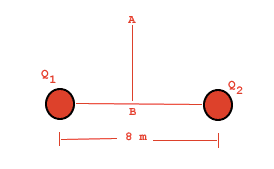# Where between two charges does voltage = 0?

## Homework StatementIf Q1 in the above figure is twice Q2 and both are positive, where can a point of zero potential be found?

V = kq/r

## The Attempt at a Solution

I know that eventually I'll have to set it up so that kq/r = kq/r, but my problem is, how do you know where this point will be? How do you know whether it will be between the two points or outside the two points? Thanks!

rcgldr
Homework Helper
If it was outside the points horizontally, then you have the sum of two forces pointing in the same direction, so it can't be outside the points. You might want to use the terms k Q1/R1 and k Q2/(8-R1).

If both charges are positive, it couldn't be between the two points either. Although there would be a point somewhere between the two charges where the force on a positively charged object would be zero, the potential would be less at some vertical distance above that point, so the potential wouldn't be zero anywhere except at an infinite distance away from both point charges.

The question was mis-worded and corrected below, so that one of the charges is negative and the other positive. In this case there would be a point where the potential is zero.

Last edited:
If it was outside the points horizontally, then you have the sum of two forces pointing in the same direction, so it can't be outside the points.

But I thought voltage was a scalar?

rcgldr
Homework Helper
But I thought voltage was a scalar?
Voltage is defined as the difference in potential per unit charge between two points, say A and B. The voltage from A to B is the negative of the voltage from B to A, so in that sense it has a direction. Link to article, look at the lower half of the page where infinity is used as a reference point:

http://hyperphysics.phy-astr.gsu.edu/hbase/electric/potpoi.html

As corrected in my previous post, there's isn't any point within finite distance of two positive point charges where the potential is zero (it can be considered to be zero at infinity).

Last edited:
SORRY for the confusion, I accidentally copied and pasted the wrong question. The actual problem is,

If Q1 is negative and twice Q2, which is positive, where will the potential be zero?

rcgldr
Homework Helper
Now it makes sense to use: k q1/(r) + k q2 / (8-r) = 0.

Last edited:
BvU